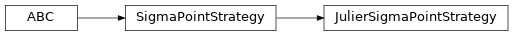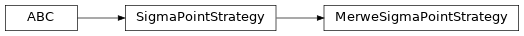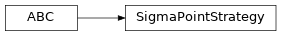# torchfilter.utils¶

## Package Contents¶

### Classes¶

 JulierSigmaPointStrategy Sigma point selection in this style of . MerweSigmaPointStrategy Sigma point selection in the style of . SigmaPointStrategy Strategy to use for computing sigma weights + selecting sigma points. UnscentedTransform Helper class for performing (batched, differentiable) unscented transforms.
class torchfilter.utils.JulierSigmaPointStrategy[source]Sigma point selection in this style of .

Keyword Arguments

lambd (Optional[float]) – Spread parameter; sometimes denoted as kappa. If None, we use 3 - dim.

lambd :Optional[float]
compute_lambda(self, dim: int) → float[source]

Compute sigma point scaling parameter.

Parameters

dim (int) – Dimensionality of input vectors.

Returns

float – Lambda scaling parameter.

compute_sigma_weights(self, dim: int) → Tuple[torch.Tensor, torch.Tensor][source]

Helper for computing sigma weights.

Parameters

dim (int) – Dimensionality of input vectors.

Returns

Tuple[torch.Tensor, torch.Tensor] – Covariance and mean weights. We expect 1D float32 tensors on the CPU.

class torchfilter.utils.MerweSigmaPointStrategy[source]Sigma point selection in the style of .

Keyword Arguments
• alpha (float) – Spread parameter. Defaults to 1e-2.

• kappa (Optional[float]) – Secondary scaling parameter, which is typically set to 0.0 or 3 - dim. If None, we use 3 - dim.

• beta (float) – Extra sigma parameter. Defaults to 2 (optimal for Gaussians, as per Section 3.2 in ).

alpha :float = 0.01
beta :float = 2.0
kappa :Optional[float]
compute_lambda(self, dim: int) → float[source]

Compute sigma point scaling parameter.

Parameters

dim (int) – Dimensionality of input vectors.

Returns

float – Lambda scaling parameter.

compute_sigma_weights(self, dim: int) → Tuple[torch.Tensor, torch.Tensor][source]

Helper for computing sigma weights.

Parameters

dim (int) – Dimensionality of input vectors.

Returns

Tuple[torch.Tensor, torch.Tensor] – Covariance and mean weights. We expect 1D float32 tensors on the CPU.

class torchfilter.utils.SigmaPointStrategy[source]

Bases: abc.ABCStrategy to use for computing sigma weights + selecting sigma points.

abstract compute_lambda(self, dim: int) → float[source]

Compute sigma point scaling parameter.

Parameters

dim (int) – Dimensionality of input vectors.

Returns

float – Lambda scaling parameter.

abstract compute_sigma_weights(self, dim: int) → Tuple[torch.Tensor, torch.Tensor][source]

Helper for computing sigma weights.

Parameters

dim (int) – Dimensionality of input vectors.

Returns

Tuple[torch.Tensor, torch.Tensor] – Covariance and mean weights. We expect 1D float32 tensors on the CPU.

class torchfilter.utils.UnscentedTransform(*, dim: int, sigma_point_strategy: SigmaPointStrategy = JulierSigmaPointStrategy())[source]

Helper class for performing (batched, differentiable) unscented transforms.

Keyword Arguments
weights_c :torch.Tensor

Unscented transform covariance weights. Note that this will be initially instantiated on the CPU, and moved in compute_distribution().

Type

torch.Tensor

weights_m :torch.Tensor

Unscented transform mean weights. Note that this will be initially instantiated on the CPU, and moved in compute_distribution().

Type

torch.Tensor

select_sigma_points(self, input_mean: torch.Tensor, input_covariance: types.CovarianceTorch) → torch.Tensor[source]

Select sigma points.

Parameters
• input_mean (torch.Tensor) – Distribution mean. Shape should be (N, dim).

• input_covariance (torch.Tensor) – Distribution covariance. Shape should be (N, dim, dim).

Returns

torch.Tensor – Selected sigma points, with shape (N, 2 * dim + 1, dim).

select_sigma_points_square_root(self, input_mean: torch.Tensor, input_scale_tril: types.ScaleTrilTorch) → torch.Tensor[source]

Select sigma points using square root of covariance.

Parameters
• input_mean (torch.Tensor) – Distribution mean. Shape should be (N, dim).

• input_scale_tril (torch.Tensor) – Cholesky decomposition of distribution covariance. Shape should be (N, dim, dim).

Returns

torch.Tensor – Selected sigma points, with shape (N, 2 * dim + 1, dim).

compute_distribution(self, sigma_points: torch.Tensor) → Tuple[torch.Tensor, types.CovarianceTorch][source]

Estimate a distribution from selected sigma points.

Parameters

sigma_points (torch.Tensor) – Sigma points, with shape (N, 2 * dim + 1, dim).

Returns

Tuple[torch.Tensor, torch.Tensor] – Mean and covariance, with shapes (N, dim) and (N, dim, dim) respectively.

compute_distribution_square_root(self, sigma_points: torch.Tensor, additive_noise_scale_tril: Optional[types.ScaleTrilTorch] = None) → Tuple[torch.Tensor, types.ScaleTrilTorch][source]

Estimate a distribution from selected sigma points; square root formulation.

Parameters
• sigma_points (torch.Tensor) – Sigma points, with shape (N, 2 * dim + 1, dim).

• additive_noise_scale_tril (torch.Tensor, optional) – Parameterizes an additive Gaussian noise term. Should be lower-trinagular, with shape (N, dim, dim).

Returns

Tuple[torch.Tensor, torch.Tensor] – Mean and square root of covariance, with shapes (N, dim) and (N, dim, dim) respectively.